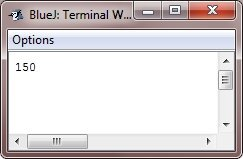# Java Number

You are free to work with numbers in Java, using basic data types, such as byte, int, double, long, etc. Here are some examples defining and initializing numbers into variables in Java:

```int numi = 432;
float numf = 43.23;```

## Java Numbers Example

Here is an example, uses numbers in Java:

```/* Java Program Example - Java Numbers */

class JavaProgram
{
public static void main(String args[])
{

Integer num = 50;
num =  num + 100;
System.out.println(num);

}
}```

Here is the output produced by the above Java program:## Examples

Here are some list of example programs, you can go for. Actually, here we listed number conversion programs.

Tools
Calculator